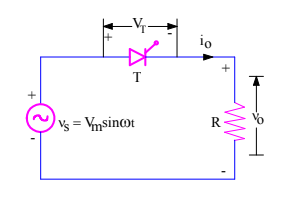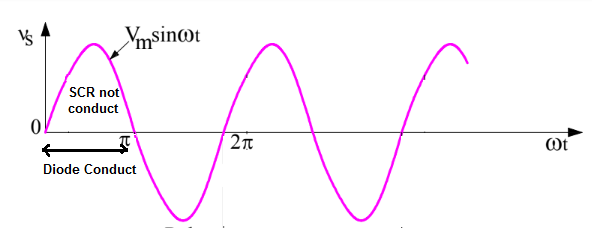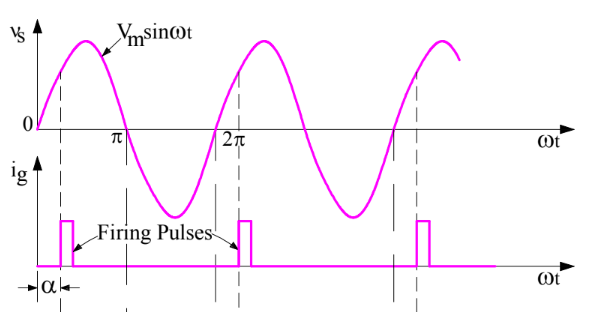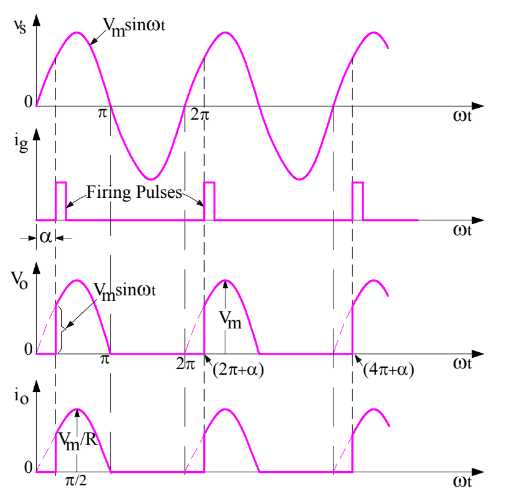# Firing Angle of SCR Explained

Firing Angle of SCR is defined as the angle between the instant SCR would conduct if it were a diode and the instant it is triggered.

We know that, there are two conditions which must be satisfied for turn on of an SCR. They are:

• SCR must be forward biased i.e. its anode voltage must be positive with respect to cathode voltage.
• It must be gated i.e. a gate signal must be applied across the Gate and Cathode Terminals.

This means, even though the SCR is forward biased, it is not going to conduct until a gate signal is applied. This is not the case with a diode. In a diode, as soon as it gets forward biased, it starts conducting. No gate signal is required to be applied for turning on a diode. In fact, there is no such Gate terminal in diode.

Let us understand the concept of firing angle in detail. For the sake of better understanding, consider the figure below.In the above figure, a thyristor T is connected to AC source vs and load resistance R. Just think, if the thyristor is replaced with a diode, it will start conducting for positive half cycle of the supply voltage as it is forward biased for this period. But will it be the same case thyristor?Obviously, No. It’s true that SCR is forward biased for positive half cycle of the supply voltage but unfortunately gate signal is not applied. Hence, it will not conduct or turn on. Let us now apply gate signal at some angle α on the source voltage curve as shown in figure below.Now, SCR is forward biased and gate signal is also applied at wt = α. Hence, SCR will turn on and will start conducting. This angle, at which gate signal is applied to SCR Gate and Cathode terminal is called Firing Angle. Application of gate signal is also called Firing of SCR. Once SCR is forward biased and fired, it becomes ON. The load voltage and current will have a wave shape similar to supply voltage as the load is resistive. What happens next? Will SCR continue to conduct for an infinite time?It’s not so. At ωt = π, the current through the SCR will become zero as the load is resistive in nature. Further, from ωt = π to 2π of supply voltage, the SCR is reversed biased. Therefore, the SCR will turn off naturally provided this half time period should be greater than SCR turn off time. This technique of SCR turn off is called Natural or Line commutation.

So, SCR will turn off at ωt = π. To turn on SCR again, we need to fire it. Therefore, we again apply gate signal at wt = (2π+α), (4π+α), (6π+α),….,so on. So there are several points where we are firing the SCR. Then what will be the firing angle?

Since, the source voltage is sinusoidal with a time period of 2π, so firing angle will be “α”.

Firing Angle may also be defined as the angle measured from the instant SCR gets forward biased to the instant it is triggered. From this definition, the firing angle for our example is α.

### One thought on “Firing Angle of SCR Explained”

1.Manju kumari siwal says:

Hm power electronic &device ko complete solution ki jurat hi polytechnic 3rd year 301 code

This site uses Akismet to reduce spam. Learn how your comment data is processed.